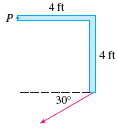# Find the magnitude of the torque

• jonnejon

## Homework Statement

Find the magnitude of the torque about P if a 68 lb force is applied as shown. Give your answer correct to the nearest integer.t=r X F

## The Attempt at a Solution

t = (4)(68)(sin30)
= 136

Don't you want to consider the distance at which it is acting is 4*√2 ?

At the point of interaction then the ⊥ component of F looks to me like F*Cos15°

## Homework Statement

Find the magnitude of the torque about P if a 68 lb force is applied as shown. Give your answer correct to the nearest integer.t=r X F

## The Attempt at a Solution

t = (4)(68)(sin30)
= 136

You wrote what must have been intended to be a vector equation for the torque, t = r x F, because the cross product only makes sense if t, r, and F are all expressed as vectors. But then you proceeded to do a scalar calculation. Why not make your torque calculation in vector form using the determinant expression for the cross product and giving the resulting torque as a vector? Then if you need the magnitude of that vector, dot it with itself and take the square root of the result.